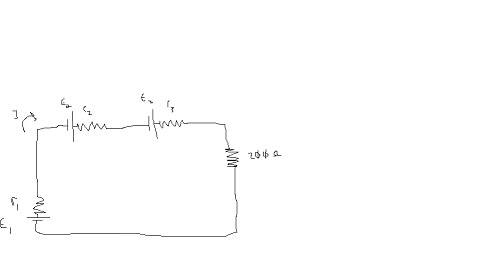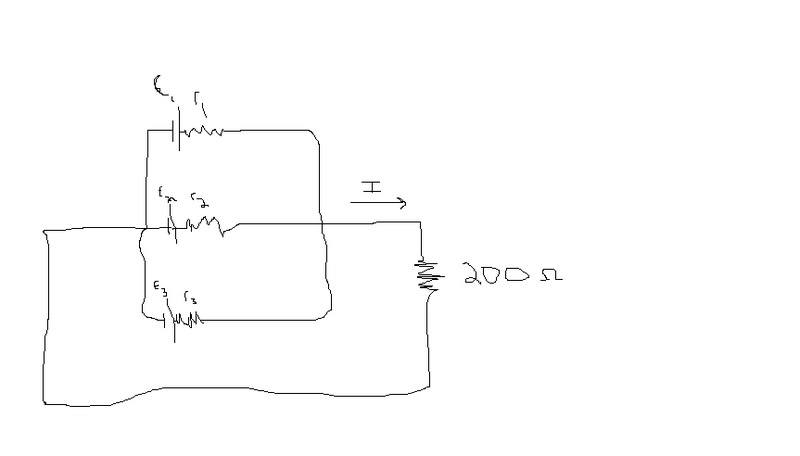# Battery Problem - With Loop Rule (pic attached)

## Homework Statement

1. "lets set the internal resistance, $$r^_i$$, of the battery with emf $$E^_i$$ equal to $$bE^_i$$ where b is a constant assumed the same for all three batteries.

$$E^_1$$=5v
$$E^_2$$=10v
$$E^_3$$=15v
r1=b$$E^_1$$
r2=b$$E^_2$$
r3=b$$E^_3$$http://i17.photobucket.com/albums/b51/chs2004/physics1.jpg

2.To decrease internal resistance we can connect the batteries in parallel, although this advantage could be offest by a decrease in net voltage. To test this hypothesis find the current flowing through the 200 ohm resistor, assume 'b' is the same from part one.http://i17.photobucket.com/albums/b51/chs2004/physics2.jpg

## Homework Equations

Kirchoffs's (sp) Loop Rule

## The Attempt at a Solution

Ok - For part one i set up the loop rule equation as follows:
$$E^_1$$-I$$r^_1$$+$$E^_2$$-I$$r^_2$$+$$E^_3$$-I$$r^_3$$-I(200ohms)=0

After substituting in the EMFs and resistances i got the equation:
-2.6=-.137b$$E^_1$$-.137b$$E^_2$$-.137b$$E^_3$$

From there i factored out the .137 and brought that to the other side and then factored out the 'b' and got:
19=b(5+10+30)
b=.6

Im not sure i did that first part right, becuase for the second part when i figure out all the currents, I1, I2, I3, they all come out to the same thing becuase the equation, I=E/R, and R=bE, the EMFs just cancel giving me 1/b for each current, 1.7A=I1=I2=I3.

Then using I=5.1A solving for the deltaV across the 200ohm resistor i get:
DeltaV=(5.1A)(200ohms)=1020V (WAYYY too high)

Im not sure where im going wrong here...i tried this and another way and i still get a deltaV higher than the combined voltages of the three batteries

I don't see how you get the first equation, or how you get from there to the second one.

I don't see how you get the first equation, or how you get from there to the second one.

i used the loop rule and started at E1 and went clockwise to get the first equation.

Then i just plugged in the values of the batteries and the resistors to solve it.

I still cannont figure out what im doing wrong becuase the voltage im getting is way out of whack.

The first equation looks wrong. What about the emf across the 200 Ohm resistor ?

The first equation looks wrong. What about the emf across the 200 Ohm resistor ?

There wouldnt be an EMF in that part of the equation for that, would there?

I thought the loop rule was, in the direction of the loop is you hit a battery - then + terminal, its positive that EMF, and when you go through a resistor if the loop and current are the same direction its -IR.

The loop doesnt go through another battery right before the 200 ohm resistor

the other way i tried the problem was like this:

using the equation: E=IR
I took the EMF of the first battery, 5v, and set the equation as follows:

5V=(.137A)(r1) ==> where r1=bE1
5V=(.137A)(5V)(b)
b=7.3

Using that value in the second part of the problem still gives me a voltage drop of 82V across that 200ohm resistor, which cant be correct.